# CAHSEE - Triangles, the Pythagorean Theorem & Congruency: Tutoring Solution Chapter Exam

Exam Instructions:

Choose your answers to the questions and click 'Next' to see the next set of questions. You can skip questions if you would like and come back to them later with the yellow "Go To First Skipped Question" button. When you have completed the practice exam, a green submit button will appear. Click it to see your results. Good luck!

### Page 1

#### Question 1 1. In the figure below, Angle 13 = 10y and Angle 11 = 3y + 11. Determine the measure of Angle 16.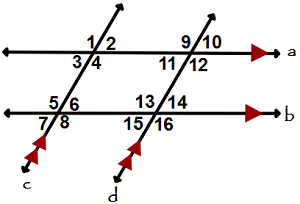#### Question 2 2. Which theorem can be used to establish congruence with the pictured triangles?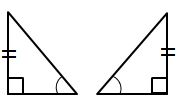#### Question 4 4. If the pictured triangles are congruent, what reason can be given?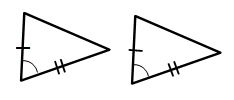### Page 2

#### Question 6 6. In triangle GBN, Angle G is seven more than three times a number, Angle B is five more than four times the number, and Angle N is twelve less than five times the number. Solve for the measure of Angle G.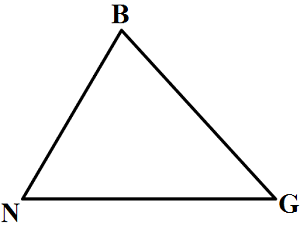#### Question 7 7. Which theorem can be used to establish congruence with the pictured triangles?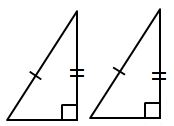#### Question 8 8. Mary claims that the triangles below are similar by AA. Do you agree or disagree with Mary?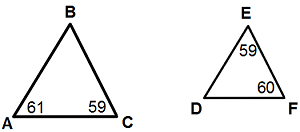#### Question 9 9. If the pictured triangles are congruent, what reason can be given?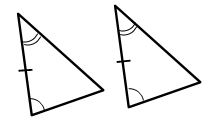### Page 3

#### Question 11 11. Determine if triangle VEZ is similar to triangle MAG.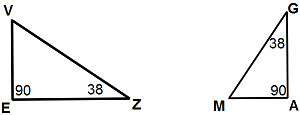#### Question 13 13. What type of triangle is pictured?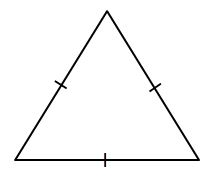#### Question 15 15. Given the triangle ABC, solve for the value of y.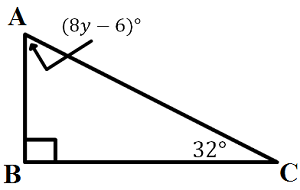### Page 4

#### Question 16 16. Which theorem can be used to establish congruence with the pictured triangles?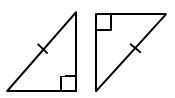#### Question 17 17. Study the given diagram. Find the value of x, the distance between T and E.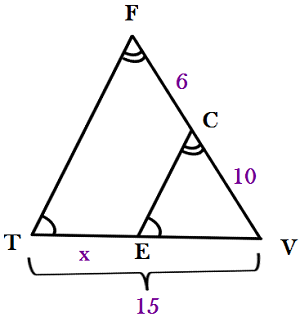#### Question 19 19. Which theorem can be used to establish congruence with the pictured triangles?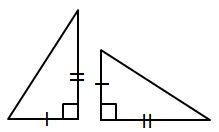#### Question 20 20. What type of triangle is pictured?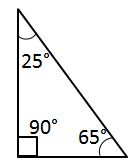### Page 5

#### Question 21 21. If the pictured triangles are congruent, what reason can be given?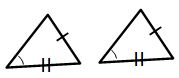#### Question 23 23. Given triangles DAR and KMR. What is the value of y?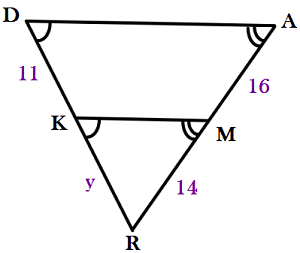#### Question 24 24. What type of triangle is pictured?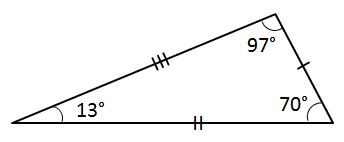#### Question 25 25. Josh claims that these triangles are similar by SSS. Do you agree or disagree with Josh?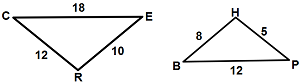### Page 6

#### Question 26 26. What type of triangle is pictured?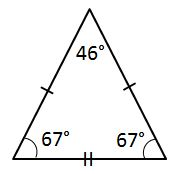#### Question 27 27. If the pictured triangles are congruent, what reason can be given?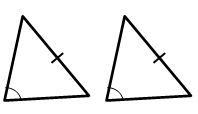#### Question 28 28. Triangle HYV and triangle AYB are similar by the AA similarity theorem. What is the value of x?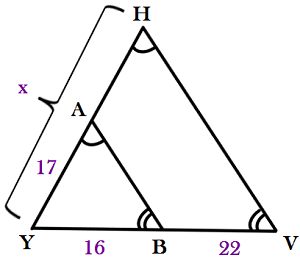#### Question 29 29. Which theorem can be used to establish congruence with the pictured triangles?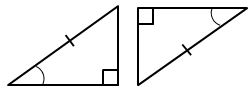#### Question 30 30. In the figure below, Angle 7 = 15x and Angle 10 = 9x + 30. Determine the measure of Angle 10.#### CAHSEE - Triangles, the Pythagorean Theorem & Congruency: Tutoring Solution Chapter Exam Instructions

Choose your answers to the questions and click 'Next' to see the next set of questions. You can skip questions if you would like and come back to them later with the yellow "Go To First Skipped Question" button. When you have completed the practice exam, a green submit button will appear. Click it to see your results. Good luck!

Support